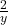## Which statement best describes the expression 3 + 2 + y? (1 point) a The quotient of y and the sum of 3 and 2 b The quotient of the sum of 3

Question

Which statement best describes the expression 3 + 2 + y? (1 point) a The quotient of y and the sum of 3 and 2 b The quotient of the sum of 3 and 2 and y с The sum of 3 and the quotient of 2 and y d The sum of 3 and the quotient of y and 2​

in progress 0
6 months 2021-09-05T00:35:32+00:00 1 Answers 0 views 0

с. The sum of 3 and the quotient of 2 and y

Step-by-step explanation:

Given that: 3 + 2 ÷ y

It would be observed that operation addition comes before division, but with respect to BODMAS; division (D) comes before addition (A).

Thus,

3 + 2  ÷ y = 3 +andis the quotient of 2 and y

Therefore, the appropriate statement that would describe the expression is; the sum of 3 and the quotient of 2 and y. This is option C in the given question.